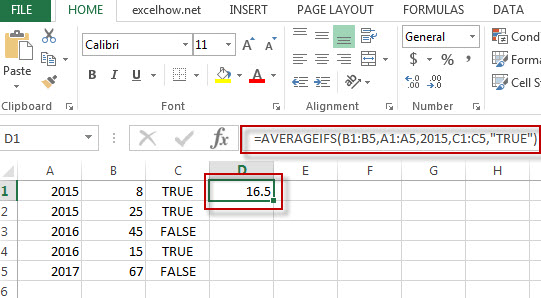# Excel AverageIFS Function

This post will guide you how to use Excel AVERAGEIFS function with syntax and examples in Microsoft excel.

### Description

The Excel AVERAGEAIFS function returns the average of all numbers in a range of cells that meet multiple criteria.

The AVERAGEIFS function is a build-in function in Microsoft Excel and it is categorized as a Statistical Function.

The AVERAGEIFS function is available in Excel 2016, Excel 2013, Excel 2010, Excel 2007, Excel 2003, Excel XP, Excel 2000, Excel 2011 for Mac.

### Syntax

The syntax of the AVERAGEIFS function is as below:

= AVERAGEIFS (average_range, criteria_range1, criteria1, [criteria_range2, criteria2],…)

Where the AVERAGEIFS function arguments are:
Average_range – This is a required argument. The range of cells that you want to average.
Criteria_range1  – This is a required argument. The range to apply the associated criteria.
Criteria – This is an optional argument. The criteria used to define which cells are averaged.

### Example

The below examples will show you how to use Excel AVERAGEIFS Function to return the average of all numbers in a range of cells based on multiple criteria.

#1 To get the average of all numbers in average range B1:B5 and meet the criteria that equal to “2015” in range A1:A5, and equal to “TRUE”in range C1:C5 , just using the following excel formula: =AVERAGEIFS(B1:B5,A1:A5,2015,C1:C5,”TRUE”)